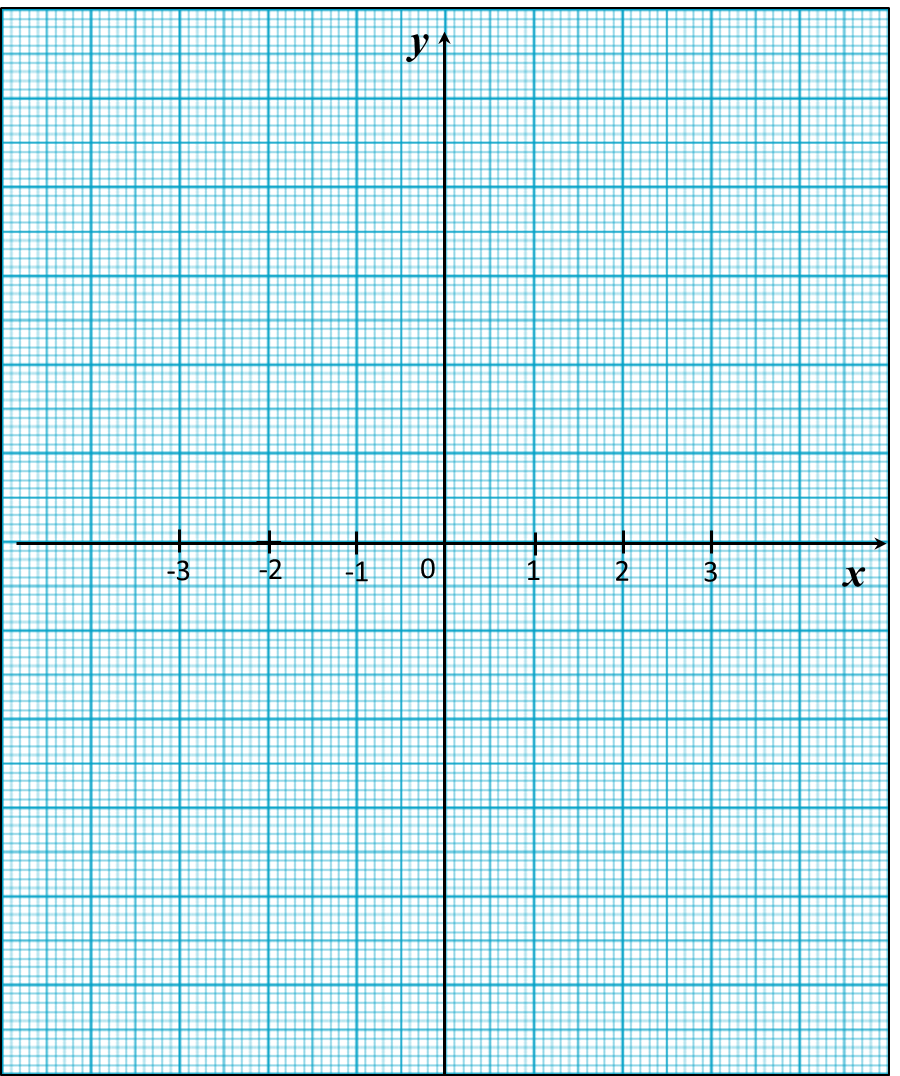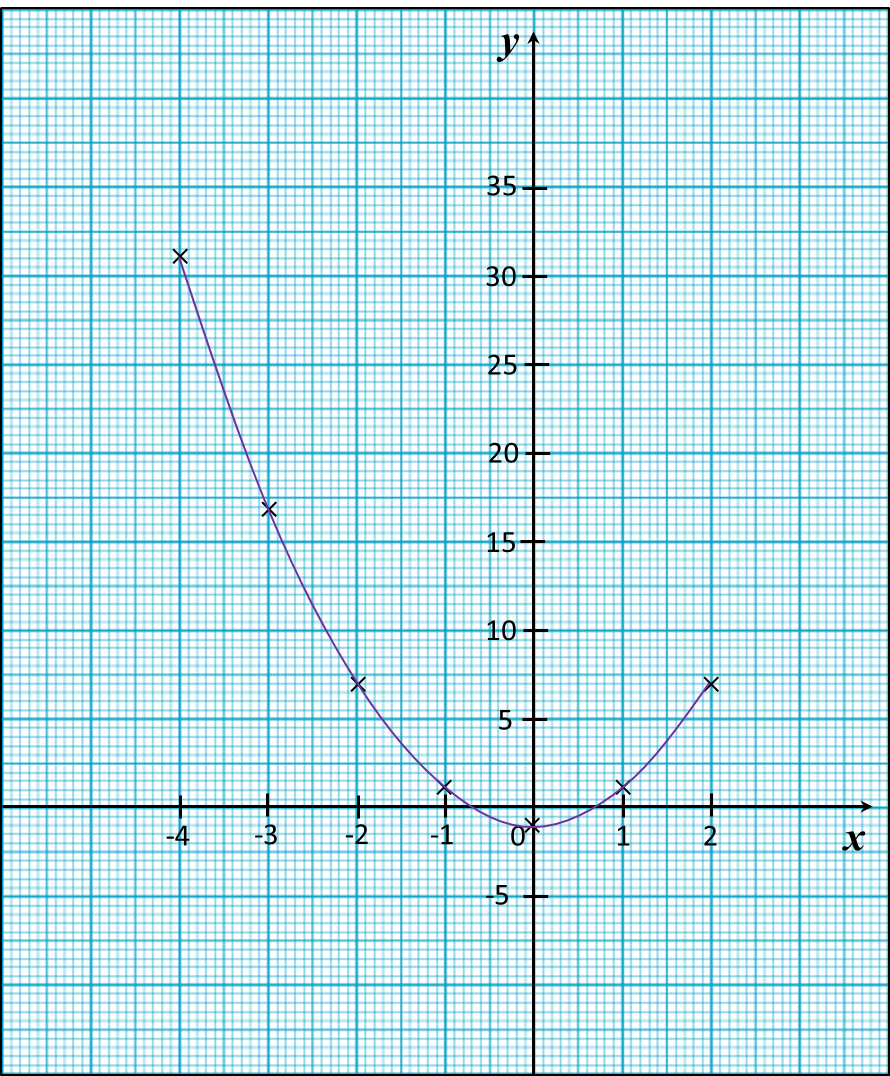# 8.2.2 Graphs of Functions, PT3 Focus Practice

         
           
               
8.2.2 Graphs of Functions, PT3 Focus Practice
                
   
  Question 4:
                
Use graph paper to answer this question.
                
Table below shows the values of two variables, x and y, of a function.
                

      
                
                 x                    –3                    –2                    –1                    0                    1                    2                    3                    y                    –19                    –3                    1                    –1                    –3                    1                    17   
                
                
The x-axis and the y-axis are provided on the graph paper on the answer space.

                
(a)  By using a scale of 2 cm to 5 units, complete and label the y-axis.
                
(b) Based on the table above, plot the points on the graph paper.
                
(c)  Hence, draw the graph of the function.
                

 
 
  
      Answer:Solution:Question 5:
                
Use graph paper to answer this question.
                
Table below shows the values of two variables, x and y, of a function.
                

      
                
                 x                    –4                    –3                    –2                    –1                    0                    1                    2                    y                    31                    17                    7                    1                    –1                    1                    7   
                
                
The x-axis and the y-axis are provided on the graph paper on the answer space.

                
(a)  By using a scale of 2 cm to 5 units, complete and label the y-axis.
                
(b)   Based on the table above, plot the points on the graph paper.
                
(c)  Hence, draw the graph of the function.Solution: